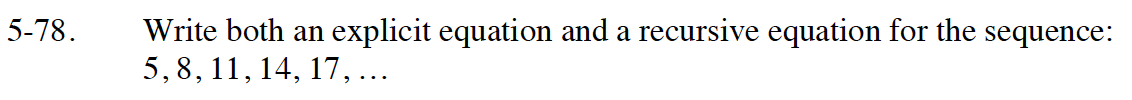Home > INT1 > Chapter Ch5 > Lesson 5.2.3 > Problem5-78

5-78.

Write both an explicit equation and a recursive equation for the sequence: Homework Help ✎
5, 8, 11, 14, 17, …Are the terms in the sequence being added to or multiplied by? In other words, is the sequence arithmetic or geometric?

Explicit: t(n) = 3n + 2
Recursive: t(1) = 5; t(n+1) = t(n) + 3